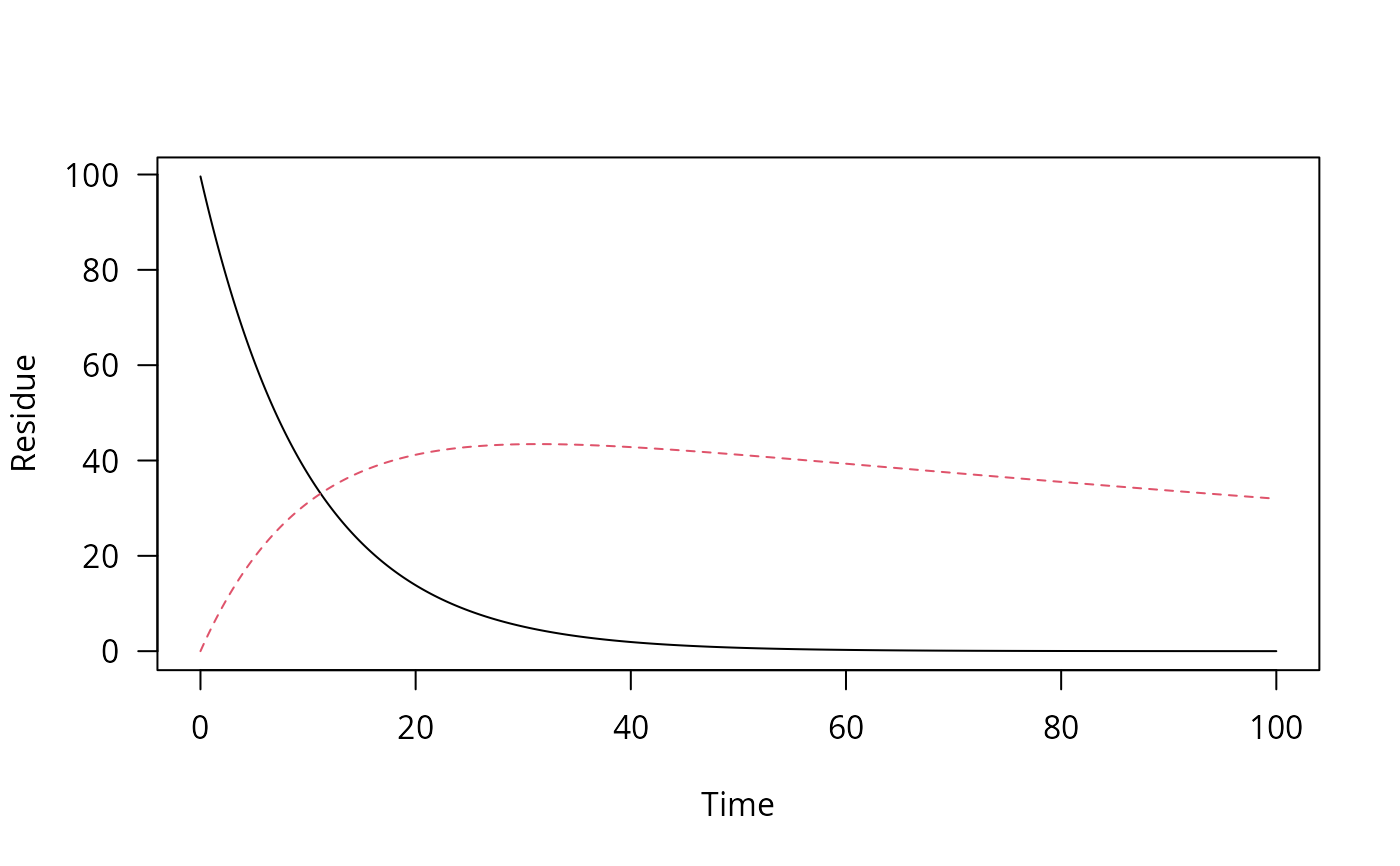Create a time series of decline data

one_box(x, ini, ..., t_end = 100, res = 0.01)

# S3 method for numeric
one_box(x, ini = 1, ..., t_end = 100, res = 0.01)

# S3 method for character
one_box(x, ini = 1, parms, ..., t_end = 100, res = 0.01)

# S3 method for mkinfit
one_box(x, ini = "model", ..., t_end = 100, res = 0.01)

## Arguments

x When numeric, this is the half-life to be used for an exponential decline. When a character string specifying a parent decline model is given e.g. FOMC, parms must contain the corresponding parameters. If x is an mkinfit object, the decline is calculated from this object. The initial amount. If x is an mkinfit object, and ini is 'model', the fitted initial concentrations are used. Otherwise, ini must be numeric. If it has length one, it is used for the parent and initial values of metabolites are zero, otherwise, it must give values for all observed variables. Further arguments passed to methods End of the time series Resolution of the time series A named numeric vector containing the model parameters

## Value

An object of class one_box, inheriting from ts.

## Examples

# Only use a half-life
pred_0 <- one_box(10)
plot(pred_0)# Use a fitted mkinfit model
require(mkin)
fit <- mkinfit("FOMC", FOCUS_2006_C, quiet = TRUE)
pred_1 <- one_box(fit)
plot(pred_1)# Use a model with more than one observed variable
m_2 <- mkinmod(parent = mkinsub("SFO", "m1"), m1 = mkinsub("SFO"))#> Successfully compiled differential equation model from auto-generated C code.fit_2 <- mkinfit(m_2, FOCUS_2006_D, quiet = TRUE)#> Warning: Observations with value of zero were removed from the datapred_2 <- one_box(fit_2, ini = "model")
plot(pred_2)